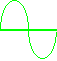e Engineer ~ Virtual ToolsEMI / EMC   Calculator~ Default Z = 50 Ω, Enter Z = 377 Ω for EM fields ~
Ω
WVdBmdBw
Copyright © Charlie Bautsch (Radioing.com).            Version 02

 Calculator Notes Electric field conversions are based on far field calculations and the characteristic impedance of free space (120π Ω or 377 Ω).  When the distance (d) from the source exceeds λ/2π, the wave impedance (ratio of the E-field to the H-field) becomes constant at 377 ohms in free space.  This is defined as the far field. Magnetic flux density (T) conversions are based on the permeability in free space (μo= 4π x 10-7 Henrys/m). H = Magnetic field strength in amps per meter (A/m). E = Electric field strength in volts per meter (V/m).  This applies to far field calculations (d > λ/2π).  Far field is generally at least 1/6 wavelengths from the antenna.  E(V/m) = (5.5*sqrt(PG))/d. P = Power (watts) into the antenna feedpoint.  This applies to antenna far field calculations using E(V/m). dB = Decibel, (10 Log10P). π = pi = 3.14159265. µ = micro = 10E-6. m = milli = 10E-3. dBw = Decibels relative to one watt. dBm = Decibels relative to one milliwatt. dBV = Decibels relative to one volt. dBµV = Decibels relative to one microvolt. dBµA = Decibels relative to one microamp. dBpT = Magnetic flux density (B) in decibels relative to one pico Tesla (pT). V = Volts. A = Amps. W = Watts. m = Meters, unit of length (39.37 inches). W/m2 = Watts per square meter (power density). mW/cm2 = Milliwatts per square centimeter (power density). V/m = Volts per meter (electric field strength). dBm/m2 = Electric field power density in decibels relative to one milliwatt per square meter. dBW/m2 = Electric field power density in decibels relative to one watt per square meter. Gain (G) = Antenna far field numeric gain relative to an isotropic radiator (antenna that radiates equally in all directions, 360 degree beamwidth). dBi = Decibels-isotropic, relative to an isotropic radiator. T = Teslas. Flux Density (T) = (Wb)/(m2). µT = MicroTeslas. Wb = Webers. Flux (Wb) = Flux Density (T) x Area (m2). A/m = Amperes per meter. Shielding (SdB) = Shielding effectiveness in decibels. Shielding effectiveness (S) is the ratio of the EMI field strength outside the shield to the field strength inside the shield. Attenuation (%Att) = Percentage attenuation of a shield.Copyright © 1997 RADIOING.com. All rights reserved.